General scarcity factor  That page is updated around 3 times/year (last: Oct 5' 2003)  The general scarcity factor is a proportion based on the distribution of the "standard-coin" H-486. For example "H-501 = 0.506" means that there are two H486 specimens for each H501 specimen. Those informations are based on the material included on  this site. . This list runs from the most "frequent" coins (H-486) to the rarest (H-428):.REFERENCE SPECIMEN: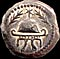H-486 =  1.000 .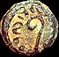H-649v =  0.937 (varieties only)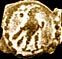H-501 =  0.506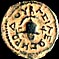H-487 =  0.310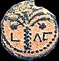H-635 =  0.291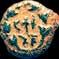H-483 =  0.277H-458 =  0.241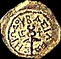H-488 =  0.234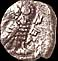H-426 =  0.215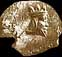H-429 =  0.196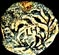H-650v= 0.190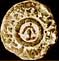H-476 =  0.184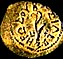H-489 =  0.171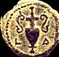H-643 =  0.171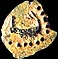H-502 =  0.171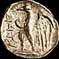H-438 =  0.146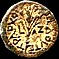H-521 =  0.114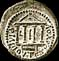H-534 =  0.108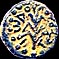H-511 =  0.108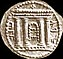H-688 =  0.101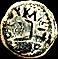H-648v =  0.101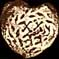H-484 =  0.101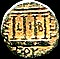H-531 =  0.095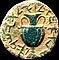H-677 =  0.089H-498 =  0.082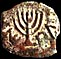H-485 =  0.082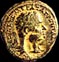H-555 =  0.076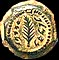H-468 =  0.076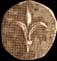H-427 =  0.063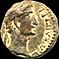H-533 =  0.057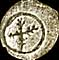H-495 =  0.044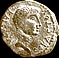H-528 =  0.044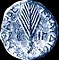H-574 =  0.044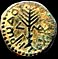H-516 =  0.044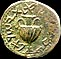H-676 =  0.044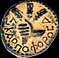H-540 =  0.044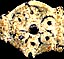H-477 =  0.044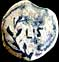H-648-B =  0.038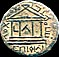H-535 =  0.038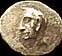H-433 =  0.038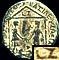H-554 =  0.038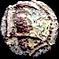H-462 =  0.038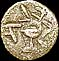H-673 =  0.032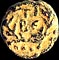H-511a =  0.032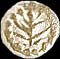H-496 =  0.025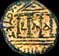H-539 =  0.013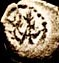H-497 =  0.013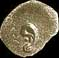H-428 =  0.013